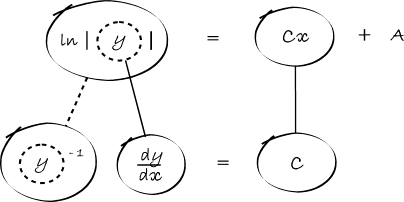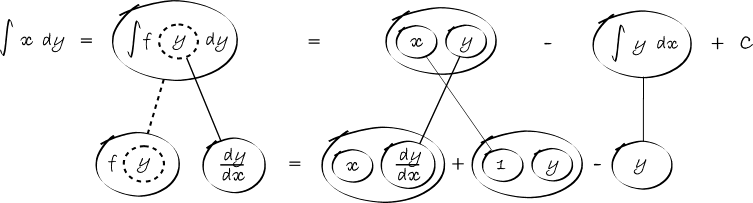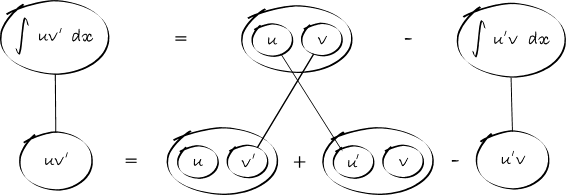# Thread: Integration Formulas and dx

1. ## Integration Formulas and dx

I am currently trying to learn about integrals, but I have run into a bit of an issue with the notation. In my calc book they give this definition for integration by parts...

$\displaystyle \int {udv = uv - \int {vdu} }$

I am able to understand why the following works and use it to do the same problems
$\displaystyle \int {u\frac{{dv}}{{dx}}dx = uv - \int {v\frac{{du}}{{dx}}} } dx$

Can someone explain why these equations are the same. If I am correct, when you examine the derivative

$\displaystyle \frac{{dy}}{{dx}}$

I know that you can define dx to be an independent variable so that

$\displaystyle dy = f'(x)*dx$

But how is this equation related to the dx in the integral. I don't seem to understand why the simplification is allowed. From what I have read, wikipedia says that the dx is related to how you define the integral (in terms of a theorem). I suppose I am using a Riemann Sum as a definition if that is what is meant.

I would simply ignore the notation and just do it my way, but in later chapters on differential equations they use differentials and integrals heavily, or so it looks like.

Thanks for any help, I have done quite a bit of work to no avail.

2. seeing as nobody is responding, if the above doesn't make any sense let me know lol.

3. I don't even bother with differentials and I already did Calc I,II,III and DE without any problems. Those differentials have something to do with some mathematical objects called forms, but unless you do theoretical math, it really doesn't matter. Just treat them like fractions and "cancel" away the denominator.

I like to keep it simple. The way I remember it is using the Product Rule. If $\displaystyle f, g$ are functions such that $\displaystyle h = f g$, then the Product Rule says $\displaystyle h' = f'g + g'f.$ The order of the terms doesn't matter as long one is a prime and the other isn't. If you now take the integral of both sides you get $\displaystyle \int h' = \int (f'g+g'f)$ and using the linear properties of the integral you get $\displaystyle \int h' = \int f'g + \int g'f$ Move whichever term you want to the other side and you get this $\displaystyle \int g'f = h - \int f'g$. Resubstitute for $\displaystyle h$ and you get the familiar formula of integration by parts. $\displaystyle \int g'f = f g - \int f'g$. Order doesn't matter as long as you keep the negative sign in the correct place.

4. Yes, we usually read

$\displaystyle \int {udv = uv - \int {vdu} }$

as

$\displaystyle \int {uv' = uv - \int {u'v} }$

where u and v are both functions of, say, x, and each integration ($\displaystyle \int$) and each differentiation ($\displaystyle '$) might be understood tacitly as being with respect to (wrt) x. In other words, we read it as shorthand for

$\displaystyle \int {u\frac{{dv}}{{dx}}dx = uv - \int {v\frac{{du}}{{dx}}} } dx$

However, I think Billa is pointing out that we can also read it as

$\displaystyle \int {u \; wrt \; v = uv - \int {v \; wrt \; u} }$

And he's asking (reasonably enough) whether we need to appeal to the cancelling of differentials (so often introduced with a health warning) in order to justify this (no doubt useful) reading.

Just suppose, for example, that someone (e.g. Balloon Calculus) had a phobia of the fractional notation, or tried to use it only interchangeably with other kinds - ruling out cancelling. Most single-variable integration is for them a matter of working backwards through the chain rule and product rule, which are taken as given. But can they justify such useful moves as

$\displaystyle \int {x \; wrt \; y = xy - \int {y \; wrt \; x} }$

?

5. Hey tom, thanks for the link and the post. I downloaded the ballooncalculus java program and it was very helpful in understanding integration by parts. I will definitely recommend it to anyone else learning about integrals.
However, I was only using integration by parts as an example. I was already able to evaluate this type of integral using a primitive version of what your program does. I guess I was mostly looking for an explanation of differentials when they are used in integration. The specific problem I was working on (which I should have posted) was this.

dy/dx = C * y

While the answer is obvious, the book works the problem in a way that I don't understand...

dy/y = c*dx
then they say they integrate both sides and get this...

ln( absolute val of (y)) = kt + C

in my understanding, there are two independent variables (in dy/y=c*dx) both x and dx. Therefore, how can you perform the operation the book does (the integration) since x and dx are unrelated? The way I think of dx is that it is an independent variable so that dy = y' * dx.

6. Hi, billa, thanks for those comments.

Actually, I did appreciate that you weren't stuck on integration by parts itself, and I thought your question about the notation of the formula was quite valid. I think the version in your book allows a reading that indicates a substitution that (no doubt usefully in many cases) avoids integration by parts - if integration by parts entails treating the integrand as a product. So the version in your book, which depends on being happy about cancelling and isolating 'differentials', is perhaps (though I'm not sure) more powerful for it.

Now you show the same approach at work in a differential equation, but here I think the choice is only a matter of taste. Their approach sets up the equation for the graceful (but for some mortals mystifying) step of 'integrating' both sides without specifying a variable of integration - merely adding a $\int$ sign on each side. So, having 'separated the variables' by turning

$\displaystyle \frac{{dy}}{{dx}} = C y$

into

$\displaystyle \frac{{1}}{{y}}dy = C dx$

they can say,

$\displaystyle \int \frac{{1}}{{y}}dy = \int C dx$

Personally, I feel more confident knowing that I'm 'balancing' by integrating each side with respect to the same variable. So I would 'separate the variables' by turning

$\displaystyle \frac{{dy}}{{dx}} = C y$

into

$\displaystyle \frac{{1}}{{y}}\frac{{dy}}{{dx}} = C$

so we can say,

$\displaystyle \int (\frac{{1}}{{y}}\frac{{dy}}{{dx}})dx = \int C dx$

- i.e. integrate both sides with respect to x. Now I grant that variables x and y are separated only EXCEPT for the presence of dx on the L.H.S. This is because the L.H.S. needs the chain-rule 'undoing', and if we are happy to undo by 'cancelling' then we reach

$\displaystyle \int \frac{{1}}{{y}}dy = \int C dx$

again. But we might be balloontegrating, instead!(Straight continuous lines integrate/differentiate (up/down) with respect to x, straight dashed lines with respect to the dashed balloon expression.)

(And: x became t, C became k, leaving C for the constant - my A?)

Hope this helps

7. PS:

Sincere apologies for posting a reply to my own question, but anyway...

Billa highlighted the fact that we can read the parts formula

$\displaystyle \int {udv = uv - \int {vdu} }$

not just in the usual way i.e.

$\displaystyle \int {uv' = uv - \int {u'v} }$

but if necessary also to do

$\displaystyle \int {u \; wrt \; v = uv - \int {v \; wrt \; u} }$

i.e.

$\displaystyle \int {x \; wrt \; y = xy - \int {y \; wrt \; x} }$

and I asked whether we need to accept cancelling of differentials to justify this move. No we don't: if y is a function of x and f is the inverse so that x = f(y), we can draw the following (interpreting as in the previous post).To someone confident with differentials this might appear a shameful waste of energy. But they might see the convenience of drawing the picture for the more usual reading, i.e. for actually doing integration by parts -some of which is, that we don't need the formula or even the substitutions u and v! E.g.

#### Search Tags

formulas, integration